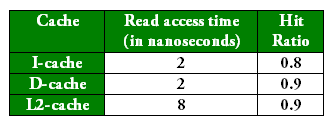# GATE | GATE CS Mock 2018 | Set 2 | Question 37

• Last Updated : 19 Nov, 2018

The read access times and the hit ratios for different caches in a memory hierarchy are as given below:The read access time of main memory in 90 nanoseconds. Assume that the caches use the referred-word-first read policy and the writeback policy. Assume that all the caches are direct mapped caches. Assume that the dirty bit is always 0 for all the blocks in the caches. In execution of a program, 60% of memory reads are for instruction fetch and 40% are for memory operand fetch. What is total value of average data fetch time multiplied by average instruction fetch time?
(A) 4.72
(B) 16.89
(C) 9.1
(D) 19.98

Explanation: Since, L2 cache is shared between Instruction and Data.

Average Instruction fetch Time = L1 access time + L1 miss rate * L2 access time + L1 miss rate * L2 miss rate * Memory access time

= 2 + 0.2 * 8 + 0.2 * 0.1 * 90
= 5.4 ns

Average Data fetch Time = L1 access time + L1 miss rate * L2 access time + L1 miss rate * L2 miss rate * Memory access time

= 2 + 0.1 * 8 + 0.1 * 0.1 * 90
= 3.7 ns

Therefore, required total value of average data fetch time multiplied by average instruction fetch time = 5.4*3.7 = 19.98

Option (D) is correct.

Quiz of this Question

My Personal Notes arrow_drop_up